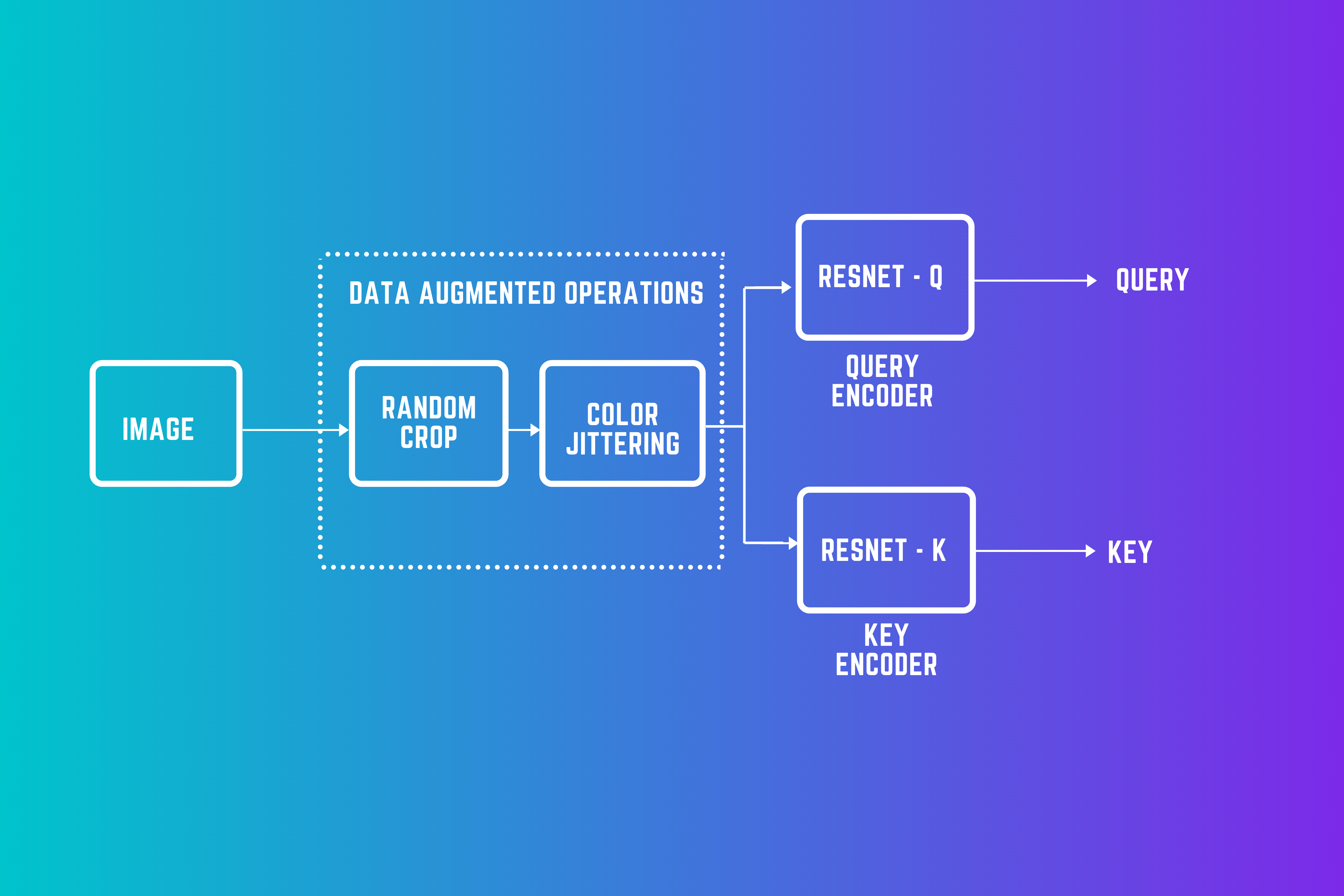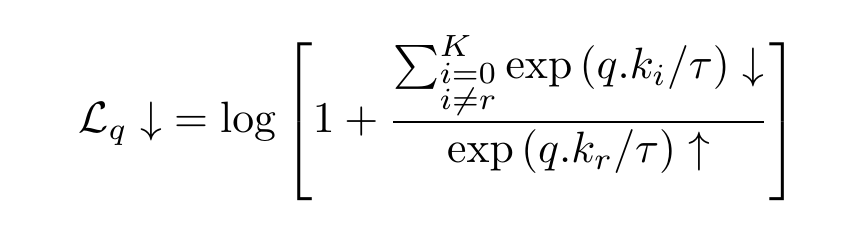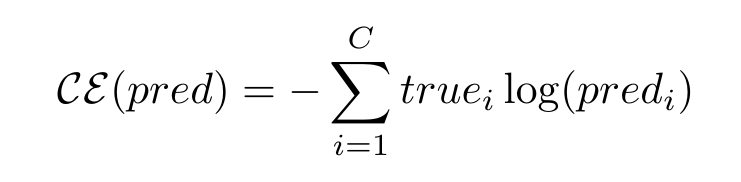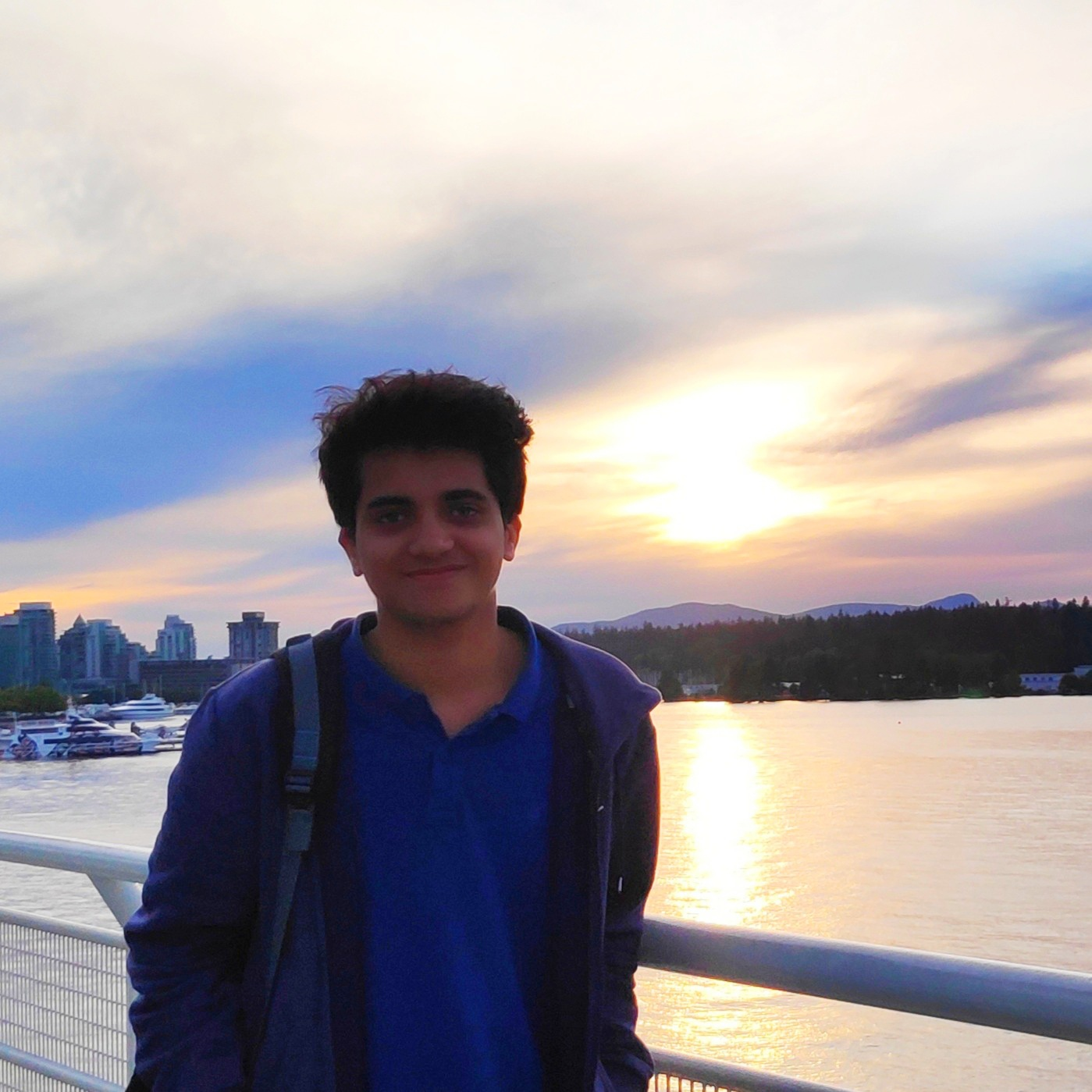Home » How to Reduce Computational Constraints using Momentum Contrast V2(Moco-v2) in PyTorch

# How to Reduce Computational Constraints using Momentum Contrast V2(Moco-v2) in PyTorch

## Introduction

The SimCLR paper explains how this framework benefits from larger models and larger batch sizes and can produce results comparable to those of supervised models if enough computing power is available. But these requirements make the framework quite computation-heavy. Wouldn’t it be wonderful if we could have the simplicity and power of this framework and have fewer compute requirements so that this can become accessible to everyone? Moco-v2 comes to the rescue.Note: In a previous blog post, we implemented the SimCLR framework in PyTorch, on a simple dataset of 5 categories with a total of just 1250 training images.

## Dataset

We will implement Moco-v2 in PyTorch on much bigger datasets this time and train our model on Google Colab. We will work with the Imagenette and Imagewoof datasets this time, made by Jeremy Howard from Fast.AI.

• Imagewoof is a dataset of 10 difficult classes from Imagenet — difficult because all classes are dog breeds. There’re a total of 9035 training, and 3939 validation set images.

## Contrastive Learning — A Review

The way contrastive learning works in self-supervised learning is based on the idea that we want different outlooks of images from the same category to have similar representations. But since we don’t know which images belong to the same category, what is generally done is that representations of different outlooks of the same image are brought closer to each other. We call these different views taken pairwise as positive pairs.

## How are negative pairs generated?

From the same image, we can get multiple representations because of random cropping. In this way, we can generate positive pairs. But how to generate negative pairs? Negative pairs are representations that come from different images. The SimCLR paper created these in the same batch. If a batch contains N images, then for each image, we get 2 representations, which accounts for a total of 2*N representations. For a particular representation x, there is one representation that forms a positive pair with x (the one that comes from the same image as x) and rest all (exactly 2*N – 2) form negative pairs with x.

### Dynamic Dictionaries

We can look at the contrastive learning approach in a slightly different way i.e., matching queries to keys. Instead of having a single encoder, we now have two encoders — one for query and another one for the key. Moreover, to have a large number of negative samples, we have a large dictionary of encoded keys.

### Challenges with this approach

• As the key encoder changes, the keys which are enqueued at later points of time can become inconsistent with the keys that were enqueued quite early. For the contrastive learning approach to work, all the keys that are compared to the queries must come from the same or similar encoders for the comparisons to be meaningful and consistent.
• Another challenge is that it’s not feasible to learn the key encoder parameters using backpropagation because that would require calculating gradients for all the samples in the queue (which would result in a large computational graph).

## The Loss Function — InfoNCE

We want a query to be close to all its positive and be far from all its negative samples. The InfoNCE loss function captures it. It stands for Information Noise Contrastive Estimation. InfoNCE loss function for a query q, for which the positive key is kᵣ is:The loss value decreases when the similarity between q and kᵣ increases and when the similarity between q and negative samples decreases
```τ = 0.05

def loss_function(q, k, queue):

# N is the batch size
N = q.shape

# C is the dimensionality of the representations
C = q.shape

# bmm stands for batch matrix multiplication
# If mat1 is a b×n×m tensor, mat2 is a b×m×p tensor,
# then output will be a b×n×p tensor.
pos = torch.exp(torch.div(torch.bmm(q.view(N,1,C), k.view(N,C,1)).view(N, 1),τ))

# performs matrix multiplication between query and queue tensors
neg = torch.sum(torch.exp(torch.div(torch.mm(q.view(N,C), torch.t(queue)),τ)), dim=1)

# sum is over positive as well as negative samples
denominator = neg + poshere predᵢ is the probability value prediction for a data point to be in the iᵗʰ class and trueᵢ is the actual probability value for that point to belong to the iᵗʰ class (which can be fuzzy, but mostly it’s one-hot).

## The MoCo-v2 Framework

Now, let’s put all the things together and see how the entire Moco-v2 Algorithm looks.

```# defining our deep learning architecture
resnetq = resnet18(pretrained=False)

classifier = nn.Sequential(OrderedDict([
('fc1', nn.Linear(resnetq.fc.in_features, 100)),
('fc2', nn.Linear(100, 50)),
('fc3', nn.Linear(50, 25))
]))

resnetq.fc = classifier
resnetk = copy.deepcopy(resnetq)

# moving the resnet architecture to device
resnetq.to(device)
resnetk.to(device)```
```# zero out grads

# retrieve xq and xk the two image batches
xq = sample_batched['image1']
xk = sample_batched['image2']

# move them to the device
xq = xq.to(device)
xk = xk.to(device)

# get their outputs
q = resnetq(xq)
k = resnetk(xk)
k = k.detach()

# normalize the ouptuts, make them unit vectors
q = torch.div(q,torch.norm(q,dim=1).reshape(-1,1))
k = torch.div(k,torch.norm(k,dim=1).reshape(-1,1))```

#### Step3:

Now, we pass our queries, keys, and the queue to our previously defined loss function and store the value in a list. Then, as usual, we call the backward function on our loss value and run the optimizer.

```# get loss value
loss = loss_function(q, k, queue)

# put that loss value in the epoch losses list
epoch_losses_train.append(loss.cpu().data.item())

# perform backprop on loss value to get gradient values
loss.backward()

# run the optimizer
optimizer.step()```
```# update the queue
queue = torch.cat((queue, k), 0)

# dequeue if the queue gets larger than the max queue size - denoted by K
# batch size is 256, can be replaced by a variable
if queue.shape > K:
queue = queue[256:,:]```
```# update resnetk
for θ_k, θ_q in zip(resnetk.parameters(), resnetq.parameters()):
θ_k.data.copy_(momentum*θ_k.data + θ_q.data*(1.0 - momentum))
```

#### Some Training Details

Training resnet-18 models took close to 18 hours of GPU time for each of the Imagenette and Imagewoof datasets. We used Google Colab’s GPU (16GB) for this purpose. We used a batch size of 256, a tau value of 0.05, a learning rate of 0.001, which we decreased eventually to 1e-5, and a weight decay of 1e-6. Our queue size was 8192 and the momentum value for the key encoder was 0.999.

## Results

The top 3 layers (treating relu as a layer) defined our projection head, which we removed for the downstream task of image classification. On top of the remaining network, we trained a linear classifier.

1. By using larger batch and dictionary sizes.
2. Using more data, if one can. Bringing in all the unlabeled data as well.
3. Training large models on large amounts of data and then distilling them.

## ReferencesYou can also read this article on our Mobile APP